## GATE 2016 [Set-1]

 Question 1

Out of the following four sentences, select the most suitable sentence with respect to grammar and usage.

 A I will not leave the place until the minister does not meet me. B I will not leave the place until the minister doesn’t meet me. C I will not leave the place until the minister meet me. D I will not leave the place until the minister meets me.
Aptitude       Verbal
Question 1 Explanation:
In option A and B, 'not' is already embedded until so both are incorrect.
Minister is a singular person, with a singular object, so verb ends with 's'. So option C is incorrect.
Option 'D' is correct.
 Question 2

A rewording of something written or spoken is a ______________.

Aptitude       verbal
Question 2 Explanation:
Paraphrase means that "express the meaning of (something written or spoken) using different words.
 Question 3

Archimedes said, “Give me a lever long enough and a fulcrum on which to place it, and I will move the world.”

The sentence above is an example of a ___________ statement.

 A figurative B collateral C literal D figurine
Aptitude       verbal
Question 3 Explanation:
Figurative means that "departing from a literal use of words"; Metaphorical.
The use of the metaphorical words to explain about the thoughts instead of literal use of them.
 Question 4

If ‘relftaga’ means carefree, ‘otaga’ means careful and ‘fertaga’ means careless, which of the following could mean ‘aftercare’?

 A zentaga B tagafer C tagazen D relffer
Aptitude       verbal
Question 4 Explanation:
relftaga - carefree → (1)
otaga - careful → (2)
fertaga - careless → (3)
From (1) & (2),
taga = care (and it is in the first half of the word)
From (3),
fer = less
Aftercare - tagazen
Care is in the second half of the word ⇒ taga should be in the first half.
 Question 5

A cube is built using 64 cubic blocks of side one unit. After it is built, one cubic block is removed from every corner of the cube. The resulting surface area of the body (in square units) after the removal is __________.

 A 56 B 64 C 72 D 96
Aptitude       Numerical
Question 5 Explanation:
64 cubic blocks are used to make the cube
⇒ Side of cube = 4
Surface area of cube = 6s2 = 6 × 42 = 96
Each corner block is associated with three faces of cube.
When they are removed, three new faces are exposed. Thus there appears no net change in the exposed surface area.
Hence, Surface area = 96
 Question 6

A shaving set company sells 4 different types of razors, Elegance, Smooth, Soft and Executive. Elegance sells at Rs. 48, Smooth at Rs. 63, Soft at Rs. 78 and Executive at Rs. 173 per piece. The table below shows the numbers of each razor sold in each quarter of a year.Which product contributes the greatest fraction to the revenue of the company in that year?

 A Elegance B Executive C Smooth D Soft
Aptitude       Numerical
Question 6 Explanation:
Revenue from Elegance = (27300 + 25222 + 28976 + 21012) × 48 = Rs. 4920480
Revenue from Smooth = (20009 + 19392 + 22429 + 18229) × 63 = Rs. 5043717
Revenue from Soft = (17602 + 18445 + 19544 + 16595) × 78 = Rs.5630508
Revenue from Executive = (9999 + 8942 + 10234 + 10109) × 173 = Rs. 6796132
Clearly, Executive contributes the greatest fraction to the revenue of the company as the revenue from it is the highest.
 Question 7

Indian currency notes show the denomination indicated in at least seventeen languages. If this is not an indication of the nation’s diversity, nothing else is.

Which of the following can be logically inferred from the above sentences?

 A India is a country of exactly seventeen languages. B Linguistic pluralism is the only indicator of a nation’s diversity. C Linguistic pluralism is the only indicator of a nation’s diversity. D Linguistic pluralism is strong evidence of India’s diversity.
Aptitude       verbal
Question 7 Explanation:
Which is inferred from the statement.
Option A, B, C are incorrect which are not properly inferred from the statement.
 Question 8

Consider the following statements relating to the level of poker play of four players P, Q, R and S.

I. P always beats Q
II. R always beats S
III. S loses to P only sometimes
IV. R always loses to Q

Which of the following can be logically inferred from the above statements?

(i) P is likely to beat all the three other players
(ii) S is the absolute worst player in the set
 A (i) only B (ii) only C (i) and (ii) D neither (i) nor (ii)
Aptitude       numerical
Question 8 Explanation:
From statements I, II & IV, we can say that
All three can beat S.
But from statement III,
S loses to P only sometimes.
(ii) Cannot be inferred.
And in poker, the transitive law does not apply. This can be seen from statement III.
As S loses to P only sometimes which states that wins against P most of the time.
So, (i) cannot be logically inferred.
 Question 9

If f(x) = 2x7 + 3x - 5, which of the following is a factor of f(x)?

 A (x 3 +8) B (x-1) C (2x-5) D (x+1)
Aptitude       Numerical
Question 9 Explanation:
f(x) = 2x7 + 3x - 5
For (x - a) to be a factor of f(x)
f(a) = 0
From options,
Only a=1, satisfies the above equation
∴ (x - 1) is a factor of f(x).
 Question 10

In a process, the number of cycles to failure decreases exponentially with an increase in load. At a load of 80 units, it takes 100 cycles for failure. When the load is halved, it takes 10000 cycles for failure. The load for which the failure will happen in 5000 cycles is ________.

 A 40 B 46.02 C 60.01 D 92.02
Aptitude       numerical
Question 10 Explanation:
In a process, the number of cycles to failure decrease exponentially with an increase in load.
General exponential function = a⋅e-bx
i.e., No. of cycles to failure = a⋅e-bx
At a load of 80 units, it takes 100 cycles to failure.
So, no. of cycles to failure = 100
i.e., 100 = a⋅e - b(80) --------(1)
When the load is halved, it takes 10000 cycles to failure.
No. of cycles to failure = 10,000
i.e., 10,000 = a⋅e - b(40) ---------(2)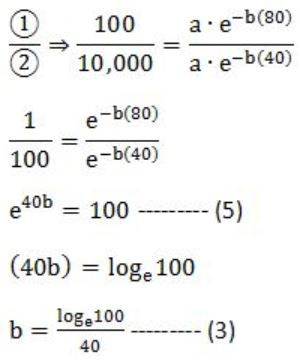No. of cycles to failure = 5,000
i.e., 5000 = a⋅e - bx
Multiply with 2 on both sides,
10,000 = 2⋅a⋅e - bx ------- (4)Question 11

Let p,q,r,s represent the following propositions.

p: x ∈ {8,9,10,11,12}
q: x is a composite number
r: x is a perfect square
s: x is a prime number

The integer x≥2 which satisﬁes ¬((p ⇒ q) ∧ (¬r ∨ ¬s))  is _________.

 A 11 B 12 C 13 D 14
Engineering-Mathematics       Prepositional-Logic
Question 11 Explanation:
Given,
~((p→q) ∧ (~r ∨ ~S))
⇒ first simplify the given statement by converging them to ∧, ∨
⇒ [~(p→q) ∨ (~(~r ∨ ~s)]
Demorgan’s law:
⇒ [~(~p ∨ q) ∨ (r ∧ s)]
∵ p→q ≡ ~p ∨ q
⇒ [(p ∧ ~q) ∨ (r ∧ s)]
p ∧ ~q is {8,9,10,11,12} ∧ {not a composite number} i.e. {11}
r ∧ s is {perfect square} ∧ {prime} i.e. no answer
So, the one and only answer is 11.
 Question 12

Let an be the number of n-bit strings that do NOT contain two consecutive 1s. Which one of the following is the recurrence relation for an?

 A an = a(n-1) + 2a(n-2) B an = a(n-1) + a(n-2) C an = 2a(n-1) + a(n-2) D an = 2a(n-1) + 2a(n-2)
Engineering-Mathematics       Combinatorics
Question 12 Explanation:
an = number of n-bit strings, that do not have two consecutive 1’s.
If n=1, we have {0,1}
# Occurrences = 2
If n=2, we have {00,01,10}
# Occurrences = 3
If n=3, we have {000,001,010,100,101}
# Occurrences = 5
It is evident that a3 = a1 + a2
Similarly, an = an-1 + an-2
 Question 13A 4 B 3 C 2 D 1
Engineering-Mathematics       Calculus
Question 13 Explanation:Question 14

A probability density function on the interval [a,1] is given by 1/x2 and outside this interval the value of the function is zero. The value of a is _________.

 A 0.7 B 0.6 C 0.5 D 0.8
Engineering-Mathematics       Probability
Question 14 Explanation:
The property of probability density function is area under curve = 1
or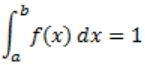where (a, b) is internal and f(x) is probability density function.
Given,
f(x) = 1/x2 , a≤x≤1
The area under curve,- 1 + 1/a = 1
1/a = 2
a = 0.5
 Question 15

Two eigenvalues of a 3 × 3 real matrix P are (2 + √-1) and 3. The determinant of P is __________.

 A 18 B 15 C 17 D 16
Engineering-Mathematics       Linear-Algebra
Question 15 Explanation:
If an eigen value of a matrix is a complex number, then there will be other eigen value, which is conjugate of the complex eigen value.
So, For the given 3×3 matrix there would be 3 eigen values.
Given eigen values are : 2+i and 3.
So the third eigen value should be 2-i.
As per the theorems, the determinant of the matrix is the product of the eigen values.
So the determinant is (2+i)*(2-i)*3 = 15.
 Question 16

Consider the Boolean operator with the following properties:Then x#y is equivalent to

 AB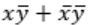C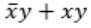DDigital-Logic-Design       Boolean-Algebra
Question 16 Explanation: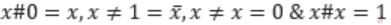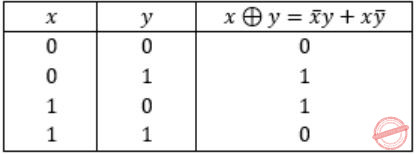Ex-OR satisfies all the properties. Hence,Question 17

The 16-bit 2’s complement representation of an integer is 1111 1111 1111 0101; its decimal representation is __________.

 A -11 B -12 C -13 D -14
Digital-Logic-Design       Number-Systems
Question 17 Explanation:
Given number is 1111 1111 1111 0101.
It is a negative number because MSB is 1.
Magnitude of 1111 1111 1111 0101 is 2’s complement of 1111 1111 1111 0101.
1111 1111 1111 0101
0000 0000 0000 1010 : 1’s Complement
0000 0000 0000 1011 : 2’s complement
= (11)10
Hence, 1111 1111 1111 0101 = -11
 Question 18

We want to design a synchronous counter that counts the sequence 0-1-0-2-0-3 and then repeats. The minimum number of J-K ﬂip-ﬂops required to implement this counter is __________.

 A 4 B 5 C 6 D 7
Digital-Logic-Design       Sequential-Circuits
Question 18 Explanation:
Given sequence is 0-1-0-2-0-3
There are 3 transitions from 0.
Hence ⌈log23⌉ = 2 bits have to be added to the existing 2 bits to represent 4 unique states.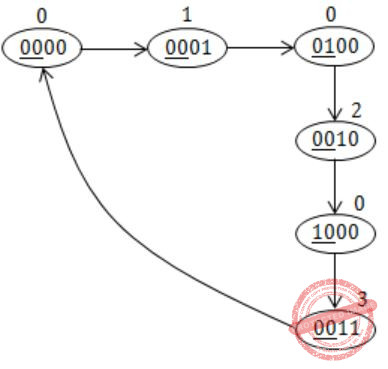Question 19

A processor can support a maximum memory of 4GB, where the memory is word-addressable (a word consists of two bytes). The size of the address bus of the processor is at least _________ bits.

 A 32 B 34 C 31 D 33
Computer-Organization       Memory-Interfacing
Question 19 Explanation:
Maximum Memory = 4GB = 232 bytes
Size of a word = 2 bytes
Therefore, Number of words = 232 / 2 = 231
So, we require 31 bits for the address bus of the processor.
 Question 20

A queue is implemented using an array such that ENQUEUE and DEQUEUE operations are performed efﬁciently. Which one of the following statements is CORRECT (n refers to the number of items in the queue)?

 A Both operations can be performed in O(1) time B At most one operation can be performed in O(1) time but the worst case time for the other operation will be Ω(n) C The worst case time complexity for both operations will be Ω(n) D Worst case time complexity for both operations will be Ω(logn)
Data-Structures       Queues
Question 20 Explanation:
Since it is mentioned in the question that both of the operations are performed efficiently.
Hence even the worst case time complexity will be O(1) by the use of the Circular queue there won't be any need of shifting in the array.
 Question 21

Consider the following directed graph: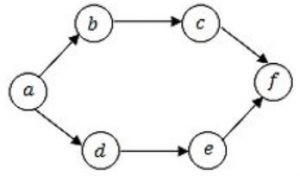The number of different topological orderings of the vertices of the graph is __________.

 A 7 B 9 C 8 D 6
Data-Structures       Topological ordering
Question 21 Explanation:
Different topological orderings of the vertices of the graph are:It is observed that (a) is the starting vertex & (f) is the final one.
Also observed that c must come after b & e must come after d.
So,Hence, there are 6 different topological orderings can be derived.
 Question 22

Consider the following C program.

```void f(int, short);
void main ()
{
int i = 100;
short s = 12;
short *p = &s;
__________ ;         // call to f()
}
```

Which one of the following expressions, when placed in the blank above, will NOT result in a type checking error?

 A f(s, *s) B i = f(i, s) C f(i, *s) D f(i, *p)
Programming       Programming
Question 22 Explanation:
int i = 100;
short s = 12;
short *p = &s;
_______ // call to f ( ) :: (void f(int,short);)
It is clearly mentioned the return type of f is void.
By doing option elimination
(A) & (C) can be eliminated as s is short variable and not a pointer variable.
(B) i = f(i, s) is false because f’s return type is void, but here shown as int.
(D) f(i, *p)
i = 100
*p = 12
Hence TRUEQuestion 23

The worst case running times of Insertion sort, Merge sort and Quick sort, respectively, are:

 A Θ(nlogn), Θ(nlogn), and Θ(n2) B Θ(n2 ), Θ(n2 ), and Θ(nlogn) C Θ(n2), Θ(nlogn), and Θ(nlogn) D Θ(n2), Θ(nlogn), and Θ(n2)
Algorithms       Sorting
Question 23 Explanation:Question 24

Let G be a weighted connected undirected graph with distinct positive edge weights. If every edge weight is increased by the same value, then which of the following statements is/are TRUE?

P: Minimum spanning tree of G does not change
Q: Shortest path between any pair of vertices does not change
 A P only B Q only C Neither P nor Q D Both P and Q
Data-Structures       Graphs
Question 24 Explanation:
Given undirected weighted graph with distinct positive edges.
Every edge weight is increased by the same value say,P: Minimum Spanning Tree will not change ABC in both the cases.
Q: Shortest path will change because in 1st figure the path from A to C calculated as ABC but in fig.2, it is AC (Direct path).
 Question 25

Consider the following C program.

```#include<stdio.h>
void mystery(int *ptra, int *ptrb) {
int *temp;
temp = ptrb;
ptrb = ptra;
ptra = temp;
}
int main() {
int a=2016, b=0, c=4, d=42;
mystery(&a, &b);
if (a < c)
mystery(&c, &a);
mystery(&a, &d);
printf("%d\n", a);
}
```

The output of the program is ________.

 A 2016 B 2017 C 2018 D 2019
Programming       Programming
Question 25 Explanation:For the first mystery (&a, &b);temp = ptr b
ptr b = ptr a
ptr a = temp
If (a The function mystery (int *ptra, int *ptrb) does not change the value of variables pointed by pointers, instead it only changes the addresses inside the pointers, which is in no way going to modify the values of a, b, c, d.
Hence, a = 2016 will be printed.
 Question 26

Which of the following languages is generated by the given grammar?

S→ aS|bS|ε
 A {anbm |n,m ≥ 0} B {w ∈ {a,b}* | w has equal number of a’s and b’s} C {an |n ≥ 0}∪{bn |n ≥ 0}∪{an b(sup>n|n ≥ 0} D {a,b}*
Theory-of-Computation       Regular-Language
Question 26 Explanation:
From the given grammar we can draw the DFA,Question 27

Which of the following decision problems are undecidable?

I. Given NFAs N1 and N2, is L(N1)∩L(N2) = Φ?
II. Given a CFG G = (N,Σ,P,S) and a string x ∈ Σ*, does x ∈ L(G)?
III. Given CFGs G1 and G2, is L(G1) = L(G2)?
IV. Given a TM M, is L(M) = Φ?
 A I and IV only B II and III only C III and IV only D II and IV only
Theory-of-Computation       Decidability-and-Undecidability
Question 27 Explanation:
Statement I is decidable, as we can make product automata by using N1 and N2 and can decide whether the resulting Product automata’s language is phi or not.
Statement II is decidable, as for CFG we have membership algorithm, hence it is decidable.
But for problems in statement III and IV, there doesn’t exist any algorithm which can decide it.
 Question 28

Which one of the following regular expressions represents the language: the set of all binary strings having two consecutive 0s and two consecutive 1s?

 A (0 + 1)* 0011(0 + 1)* + (0 + 1)* 1100(0 + 1)* B (0 + 1)* (00(0 + 1)* 11 + 11(0 + 1)* 00)(0 + 1)* C (0 + 1)* 00(0 + 1)* + (0 + 1)* 11(0 + 1)* D 00(0 + 1)* 11 + 11(0 + 1)* 00
Theory-of-Computation       Regular-Expressions
Question 28 Explanation:
Option A doesn’t generate string “001011” as it has two consecutive 0’s and two consecutive 1’s.
Option C generates string “00” which doesn’t have two consecutive 1’s.
Option D doesn’t generate string “00110” which has two consecutive 0’s and two consecutive 1’s.
 Question 29

Consider the following code segment.

x = u - t;
y = x * v;
x = y + w;
y = t - z;
y = x * y;

The minimum number of  variables required to convert the above code segment to static single assignment form is ________.

 A 10 B 11 C 12 D 13
Compiler-Design       Static-single-assignment
Question 29 Explanation:
In Static Single Assignment form (SSA) each assignment to a variable should be specified with distinct names.
Generally, subscripts are used to distinguish each definition of variables.
In the given code segment, there are two assignments of the variable x
x = u - t;
x = y + w;
and three assignments of the variable y.
y = x * v;
y = t - z;
y = x * y
Hence, two variables viz x1, x2 should be used for specifying distinct assignments of x
and for y it is named as y1, y2 and y3 for each assignment of y.
Hence, total number of variables is 10 (x1, x2, y1, y2, y3, t, u, v, w, z), and there are 5 temporary variables.
Static Single Assignment form (SSA) of the given code segment is:
x1 = u - t;
y1 = x1 * v;
x2 = y1 + w;
y2 = t - z;
y3 = x2 * y2;
 Question 30

Consider an arbitrary set of CPU-bound processes with unequal CPU burst lengths submitted at the same time to a computer system. Which one of the following process scheduling algorithms would minimize the average waiting time in the ready queue?

 A Shortest remaining time ﬁrst B Round-robin with time quantum less than the shortest CPU burst C Uniform random D Highest priority ﬁrst with priority proportional to CPU burst length
Operating-Systems       Process-Scheduling
Question 30 Explanation:
From the above question, we can get the following information.
We can consider an arbitrary set of CPU-bound processes with unequal CPU burst lengths submitted at the same time to a computer system.
We have to choose the appropriate process scheduling algorithms, which would minimize the average waiting time in the ready queue.
Waiting time is the time for which process is ready to run but not executed by CPU scheduler.
In all CPU Scheduling algorithms, shortest job first is optimal.
It gives minimum turnaround time, minimum average waiting time and high throughput and the most important thing is that shortest remaining time first is the preemptive version of shortest job first.
This scheduling algorithm may lead to starvation because if the short processes are added to the CPU scheduler continuously then the currently running process will never be able to execute as they will get pre-empted but here all the processes are arrived at same time so there will be no issue such as starvation.
SRTF would be same as SJF.
So, A is the answer. Shortest remaining time first.
 Question 31

Which of the following is NOT a superkey in a relational schema with attributes V, W, X, Y, Z and primary key VY?

 A VXYZ B VWXZ C VWXY D VWXYZ
Database-Management-System       Normalization
Question 31 Explanation:
It is given that “VY” is a primary key of the relational schema.
Any superset of “VY” is a super key. So, option (B) does not contain “Y”.
 Question 32

NOT a part of the ACID properties of database transactions?

 A Atomicity B Consistency C Isolation D Deadlock-freedom
Database-Management-System       Transactions
Question 32 Explanation:
A transaction in a database system must maintain Atomicity, Consistency, Isolation and Durability – commonly known as ACID properties.
So, Deadlock – freedom is not there in the ACID properties.
 Question 33

A database of research articles in a journal uses the following schema.

(VOLUME, NUMBER, STARTPAGE, ENDPAGE, TITLE, YEAR, PRICE)

The primary key is (VOLUME, NUMBER, STARTPAGE, ENDPAGE) and the following functional dependencies exist in the schema.

(VOLUME, NUMBER, STARTPAGE, ENDPAGE) → TITLE
(VOLUME, NUMBER) → YEAR
(VOLUME, NUMBER, STARTPAGE, ENDPAGE) → PRICE

The database is redesigned to use the following schemas.

(VOLUME, NUMBER, STARTPAGE, ENDPAGE, TITLE, PRICE)
(VOLUME, NUMBER, YEAR)

Which is the weakest normal form that the new database satisﬁes, but the old one does not?

 A 1NF B 2NF C 3NF D BCNF
Database-Management-System       Normalization
Question 33 Explanation:
Journal (V, N, S, E, T, Y, P)
V – VOLUME
N – NUMBER
S – STARTPAGE
E – ENDPAGE
T – TITLE
Y – YEAR
P – PRICE
Primary key: (V, N, S, E)
FD set:
(V, N, S, E) → T
(V, N) → Y
(V, N, S, E) → P
In (V, N) → Y; V, N is a part of the key and Y is non-prime attribute.
So, it is a partial dependency.
Now, the schema “Journal” is in 1NF but not in 2NF.
The database is redesigned as follows:Both R1 and R2 are in BCNF.
Therefore, 2NF is the weakest normal form that the new database satisfies, but the old one does not.
 Question 34

Which one of the following protocols is NOT used to resolve one form of address to another one?

 A DNS B ARP C DHCP D RARP
Computer-Networks       Protocols
Question 34 Explanation:
DHCP is dynamic host configuration protocol: allocates one of the unused IP address.
Except DHCP, remaining all the protocols are used to resolve one form of address to another one.
I. DNS is going to convert hostname to IP address.
II. ARP is going to convert IP to MAC.
III. DHCP is going to assign IP dynamically.
IV. RARP is going to convert MAC to IP.
 Question 35

Which of the following is/are example(s) of stateful application layer protocols?

(i) HTTP
(ii) FTP
(iii) TCP
(iv) POP3
 A (i) and (ii) only B (ii) and (iii) only C (ii) and (iv) only D (iv) only
Computer-Networks       Application-Layer-Protocol
Question 35 Explanation:
Stateless protocol is a communications protocol in which no information is retained by either sender or receiver.
A protocol that requires keeping of the internal state on the server is known as a stateful protocol.
Stateless - HTTP, IP
Stateful - FTP, SMTP, POP3, TCP
TCP is stateful as it maintains connection information across multiple transfers, but TCP is a Transport layer protocol.
FTP and POP3 is stateful Application layer protocol.
 Question 36

The coefﬁcient of x12 in (x3 + x4 + x5 + x6 + ...)3 is _________.

 A 10 B 11 C 12 D 13
Engineering-Mathematics       Combinatorics
Question 36 Explanation:
Co-efficient of x12 in (x3 + x4 + x5 + x6+...)3
⇒ [x3(1 + x + x2 + x3 + ...)]3
= x9(1 + x + x2 + x3 + ...)3
First Reduction:
As x9 is out of the series, we need to find the co-efficient of x3 in (1 + x + x2 + ⋯)3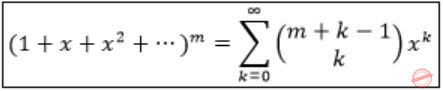Here, m=3, k=3, the coefficient= 5C3 = 5!/2!3! = 10
 Question 37

Consider the recurrence relation a1 = 8, an = 6n2 + 2n + an-1. Let a99 = K × 104. The value of K is ___________.

 A 198 B 199 C 200 D 201
Engineering-Mathematics       Combinatorics
Question 37 Explanation:
an = 6n2 + 2n + a(n-1)
Replace a(n-1)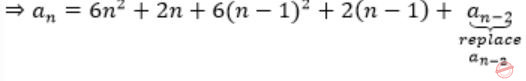⇒ an = 6n2 + 2n + 6(n-1)2 + 2(n-1) + 6(n-2)2 + 2(n-2) + ⋯ a1
Given that a1 = 8, replace it
⇒ an = 6n2 + 2n + 6(n-1)2 + 2(n-1) + 6(n-2)2 + 2(n-2) + ⋯8
= 6n2 + 2n + 6(n-1)2 + 2(n-1) + 6(n-2)2 + 2(n-2) + ⋯ + 6(1)2 + 2(1)= 6(n2 + (n-1)2 + (n-2)2 + ⋯ + 22 + 12) + 2(n + (n-1) + ⋯1)
Sum of n2 = (n(n+1)(2n+1))/6
Sum of n = (n(n+1))/2
= 6 × (n(n+1)(2n+1))/6 + 2×(n(n+1))/2
= n(n+1)[1+2n+1]
= n(n+1)[2n+2]
= 2n(n+1)2
Given a99 = k×104
a99 = 2(99)(100)2 = 198 × 104
∴k = 198
 Question 38

A function f:N+ → N+, deﬁned on the set of positive integers N+, satisﬁes the following properties:

f(n) = f(n/2)           if n is even
f(n) = f(n+5)           if n is odd

Let R = {i|∃j: f(j)=i} be the set of distinct values that f takes. The maximum possible size of R is __________.

 A 2 B 3 C 4 D 5
Engineering-Mathematics       Set-Theory
Question 38 Explanation:
f(n)= f(n⁄2)          if n is even
f(n)= f(n+5)        if n is oddWe can observe thatand f(5) = f(10) = f(15) = f(20)
Observe that f(11) = f(8)
f(12) = f(6) = f(3)
f(13) = f(9) = f(14) = f(7) = f(12) = f(6) = f(3)
f(14) = f(9) = f(12) = f(6) = f(3)
f(16) = f(8) = f(4) = f(2) = f(1) [repeating]
So, we can conclude that
‘R’ can have size only ‘two’ [one: multiple of 5’s, other: other than 5 multiples]
 Question 39

Consider the following experiment.

Step1. Flip a fair coin twice.
Step2. If the outcomes are (TAILS, HEADS) then output Y and stop.
Step4. If the outcomes are (TAILS, TAILS), then go to Step 1.

The probability that the output of the experiment is Y is (up to two decimal places) ________.

 A 0.33 B 0.34 C 0.35 D 0.36
Engineering-Mathematics       Probability
Question 39 Explanation:
If a coin is flipped twice, the possible outcomes {HH, HT, TH, TT}
Stop conditions:
If outcome = TH then Stop [output 4] --------------- (1)
else
outcome = HH/ HT then Stop [output N] -------------- (2)
We get ‘y’ when we have (1) i.e., ‘TH’ is output.
(1) can be preceded by ‘TT’ also, as ‘TT’ will reset (1) again
Probability of getting y = TH + (TT)(TH) + (TT)(TT)(TH) + …
= 1/2 × 1/2 + 1/2 × 1/2 × 1/2 × 1/2 + ...
= (1/4)/(1-1/4)
= 1/3
= 0.33
 Question 40

Consider the two cascaded 2-to-1 multiplexers as shown in the ﬁgure.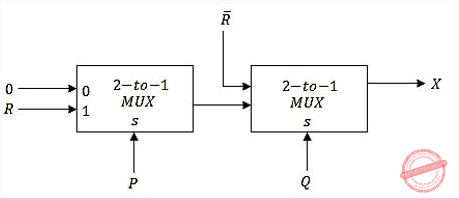The minimal sum of products form of the output X is

 ABC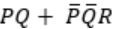DDigital-Logic-Design       Multiplexer
Question 40 Explanation:
Output of 1st MUX isNowQuestion 41

The size of the data count register of a DMA controller is 16 bits. The processor needs to transfer a ﬁle of 29,154 kilobytes from disk to main memory. The memory is byte addressable. The minimum number of times the DMA controller needs to get the control of the system bus from the processor to transfer the ﬁle from the disk to main memory is ________.

 A 456 B 457 C 458 D 459
Computer-Organization       DMA
Question 41 Explanation:
Since nothing is mentioned about the mode of DMA working whether it is cycle stealing mode or burst mode, we consider it as burst mode by default.
As the data count register of the DMA is 16 bits long in burst mode DMA transfers 216 Bytes (= 64KB) once it gets the control.
To transfer 29,154 KB, no. of times DMA needs to take control
= (29,154 KB / 64KB)
= 29,154/64
= 455.53, means 456 times.
 Question 42

The stage delays in a 4-stage pipeline are 800, 500, 400 and 300 picoseconds. The ﬁrst stage (with delay 800 picoseconds) is replaced with a functionally equivalent design involving two stages with respective delays 600 and 350 picoseconds. The throughput increase of the pipeline is ________ percent.

 A 33.33% B 33.34% C 33.35% D 33.36%
Computer-Organization       Pipelining
Question 42 Explanation:
In a pipelined processor the throughput is 1/clock cycle time.
Cycle time = max of all stage delays.
In the first case max stage delay = 800.
So throughput = 1/800 initially.
After replacing this stage with two stages of delays 600, 350... the cycle time = maximum stage delay = 600.
So the new throughput = 1/600.
The new throughput > old throughput.
And the increase in throughput = 1/600 - 1/800.
We calculate the percentage increase in throughput w.r.t initial throughput, so the % increase in throughput
= (1/600 - 1/800) / (1/800) * 100
= ((800 / 600) - 1) * 100
= ((8/6) -1) * 100
= 33.33%
 Question 43

Consider a carry lookahead adder for adding two n-bit integers, built using gates of fan-in at most two. The time to perform addition using this adder is __________.

 A Θ(1) B Θ(log(n)) C Θ(√n) D Θ(n)
Question 43 Explanation:
Formula: θ(logk (n))
Where n is number of bits added
and k is fan-in of the gates.
As we are adding n-bit numbers and fan-in is at most 2,
the solution is θ(log2 (n)).
 Question 44

The following function computes the maximum value contained in an integer array p[] of size n (n >= 1).

```       int max(int *p, int n) {
int a=0, b=n-1;

while (__________) {
if (p[a] <= p[b]) {a = a+1;}
else                 {b = b-1;}
}

return p[a];
}
```

The missing loop condition is

 A a != n B b != 0 C b > (a + 1) D b != a
Programming       Programming
Question 44 Explanation:
main ( )
{
int arr [ ] = {3, 2, 1, 5, 4};
int n = sizeof(arr) / sizeof (arr);
printf (max(arr, 5));
}
int max (int *p, int n)
{
int a = 0, b = n – 1;
(while (a!=b))
{
if (p[a] <= p[b])
{
a = a + 1;
}
else
{
b =b – 1;
}
}
return p[a];
}
The function computes the maximum value contained in an integer array p[ ] of size n (n >= 1).
If a = = b, means both are at same location & comparison ends.
 Question 45

What will be the output of the following C program?

```void count(int n)  {
static int d=1;

printf("%d ", n);
printf("%d ", d);
d++;
if(n>1) count(n-1);
printf("%d ", d);
}

void main() {
count(3);
}
```
 A 3 1 2 2 1 3 4 4 4 B 3 1 2 1 1 1 2 2 2 C 3 1 2 2 1 3 4 D 3 1 2 1 1 1 2
Programming       Programming
Question 45 Explanation:Count (3)
static int d = 1
It prints 3, 1
d++; //d = 2
n>1, count(2)
prints 2, 2
d++; // d = 3
n>1, count(1)
prints 1, 3 → Here n = 1, so condition failed & printf (last statement) executes thrice & prints d
d++; //d=4 value as 4. For three function calls, static value retains.
∴ 312213444
 Question 46

What will be the output of the following pseudo-code when parameters are passed by reference and dynamic scoping is assumed?

```       a=3;
void n(x) {x = x * a; print(x);}
void m(y) {a = 1; a = y - a; n(a); print(a);}
void main() {m(a);}
```
 A 6, 2 B 6, 6 C 4, 2 D 4, 4
Programming       Programming
Question 46 Explanation:First m(a) is implemented, as there are no local variables in main ( ), it takes global a = 3;
m(3) is passed to m(y).
a = 1
a = 3 – 1 = 2
n(2) is passed to n(x).
Since it is dynamic scoping
x = 2 * 2 = 4 (a takes the value of its calling function not the global one).
The local x is now replaced in m(y) also.
Hence, it prints 4,4.
And we know it prints 6, 2 if static scoping is used.
It is by default in C programming.
 Question 47

An operator delete(i) for a binary heap data structure is to be designed to delete the item in the i-th node. Assume that the heap is implemented in an array and i refers to the i-th index of the array. If the heap tree has depth d (number of edges on the path from the root to the farthest leaf), then what is the time complexity to re-ﬁx the heap efﬁciently after the removal of the element?

 A O(1) B O(d) but not O(1) C O(2d) but not O(d) D O(d 2d) but not O(2d)
Data-Structures       Binary-Heap
Question 47 Explanation:
→ If heap has n elements generally it takes O(n) to delete any arbitrary element.
→ Because we first need to find that element, O(n) time
Delete that element O(height) [deleting involves swapping particular element to rightmost leaf and then do heapify on that node].
**but here, we don't need to find that element, because in delete(i), i is index of that element.
Note: Delete time = O(height) = O(d)
 Question 48

Consider the weighted undirected graph with 4 vertices, where the weight of edge {i,j} is given by the entry Wij  in the matrix W.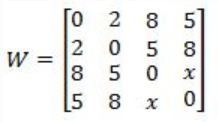The largest possible integer value of x, for which at least one shortest path between some pair of vertices will contain the edge with weight x is _________.

 A 12 B 13 C 14 D 15
Data-Structures       Graphs
Question 48 Explanation:
Let vertices be A, B, C and D.
x directly connects C to D.
The shortest path (excluding x) from C to D is of weight 12 (C-B-A-D).
 Question 49

Let G be a complete undirected graph on 4 vertices, having 6 edges with weights being 1, 2, 3, 4, 5, and 6. The maximum possible weight that a minimum weight spanning tree of G can have is __________.

 A 7 B 8 C 9 D 10
Algorithms       Minimum-Spanning-Tree
Question 49 Explanation:
Let G be a complete undirected graph with 4 vertices & 6 edges so according to graph theory, if we use Prim’s / Kruskal’s algorithm, the graph looks like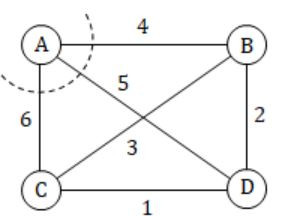Now consider vertex A to make Minimum spanning tree with Maximum weights.
As weights are 1, 2, 3, 4, 5, 6. AB, AD, AC takes maximum weights 4, 5, 6 respectively.
Next consider vertex B, BA = 4, and minimum spanning tree with maximum weight next is BD & BC takes 2, 3 respectively.
And the last edge CD takes 1.
So, 1+2+4 in our graph will be the Minimum spanning tree with maximum weights.
 Question 50

G = (V,E) is an undirected simple graph in which each edge has a distinct weight, and e is a particular edge of G. Which of the following statements about the minimum spanning trees (MSTs) of G is/are TRUE?

I. If e is the lightest edge of some cycle in G, then every MST of G includes e
II. If e is the heaviest edge of some cycle in G, then every MST of G excludes e
 A I only B II only C both I and II D neither I nor II
Algorithms       Minimum-Spanning-Tree
Question 50 Explanation:
Statement-1: False
The MSTs of G may or may not include the lightest edge.
Take rectangular graph labelled with P,Q,R,S.
Connect with P-Q = 5, Q-R = 6, R-S = 8, S-P = 9, P-R = 7.
When we are forming a cycle R-S-P-R. P-R is the lightest edge of the cycle.
The MST abcd with cost 11
P-Q + Q-R + R-S does not include it.
Statement-2: True
Suppose there is a minimum spanning tree which contains e. If we add one more edge to the spanning tree we will create a cycle.
Suppose we add edge e to the spanning tree which generated cycle C.
We can reduce the cost of the minimum spanning tree if we choose an edge other than e from C for removal which implies that e must not be in minimum spanning tree and we get a contradiction.
 Question 51

Let Q denote a queue containing sixteen numbers and S be an empty stack. Head(Q) returns the element at the head of the queue Q without removing it from Q. Similarly Top(S) returns the element at the top of S without removing it from S. Consider the algorithm given below.The maximum possible number of iterations of the while loop in the algorithm is _________.

 A 256 B 257 C 258 D 259
Data-Structures       Queues-and-Stacks
Question 51 Explanation:
The maximum possible number of iterations of the while loop in this algorithm is:
Try to solve it for 3 numbers [1. 2, 3].
Step 1: Initially Queue contains 3 elements so after 5 while loop iterations queue contains 3, 2 and stack contains 1.
Step 2: Now after 3 more while loop iterations, Queue contains 3 and stack contains 1, 2 (TOS = 2).
Step 3: After 1 more while loop iteration, push 3 onto the stack so queue is empty and stack contains 1, 2, 3 {top = 3}.
So, total number of iterations will be 5 + 3 + 1 = 9
i.e., for 3 it is 9 iterations (3*3)
for 4 it is 16 iterations (4*4)
Given: 16 numbers, so 16 * 16 = 256
 Question 52

Consider the following context-free grammars:

```G1: S → aS|B, B → b|bB
G2: S → aA|bB, A → aA|B|ε, B → bB|ε
```

Which one of the following pairs of languages is generated by G1 and G2, respectively?

 A {am bn│m > 0 or n > 0} and {am bn |m > 0 and n > 0} B {am bn│m > 0 and n > 0} and {am bn |m > 0 or n≥0} C {am bn│m≥0 or n > 0} and {am bn |m > 0 and n > 0} D {am bn│m≥0 and n > 0} and {am bn |m > 0 or n > 0}
Theory-of-Computation       Membership-Function
Question 52 Explanation:
G1:
S→aS;
will generate any number of a’s and then we can have any number of b’s (greater than zero) after a’s by using he productions
S→B and B→b|bB
G2:
By using S→aA and then A→aA | ϵ we can have only any number of a’s (greater than zero) OR we can use A→B and B→bB | ϵ to add any number of b’s after a’s OR by using S→bB and B→bB | ϵ we can have only any number of b’s (greater than zero).
 Question 53

Consider the transition diagram of a PDA given below with input alphabet Σ = {a,b} and stack alphabet Γ = {X,Z}. Z is the initial stack symbol. Let L denote the language accepted by the PDA.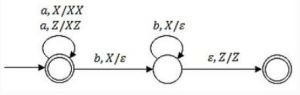Which one of the following is TRUE?

 A L = {an bn│n ≥ 0} and is not accepted by any ﬁnite automata B L = {an |n≥0} ∪ {anbn|n ≥ 0} and is not accepted by any deterministic PDA C L is not accepted by any Turing machine that halts on every input D L = {an |n ≥ 0} ∪ {an bn |n ≥ 0} and is deterministic context-free
Theory-of-Computation       Push-Down-Automata
Question 53 Explanation:
In this PDA, we can give labels to state as q0, q1, q2
where q0 and q2 are final states.
This PDA accepts the string by both ways i.e. by using q0 accepts as final state and by using q2 it accepts as empty stack.
Since q0 is initial as well as final state, so it can accept any number of a’s (including zero a’s) and by using q2 as empty stack it accept strings which has equal number of a’s and b’s (b’s comes after a’s).
Hence, L = {an | n≥0} ∪ { an bn | n≥0}.
 Question 54

Let X be a recursive language and Y be a recursively enumerable but not recursive language. Let W and Z be two languages such that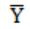reduces to W, and Z reduces to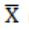(reduction means the standard many-one reduction). Which one of the following statements is TRUE?

 A W can be recursively enumerable and Z is recursive. B W can be recursive and Z is recursively enumerable. C W is not recursively enumerable and Z is recursive. D W is not recursively enumerable and Z is not recursive.
Theory-of-Computation       Recursive-Enumerable-Languages
Question 54 Explanation:
The rules are:
If A ≤ p B
Rule 1: If B is recursive then A is recursive
Rule 2: If B is recursively enumerable then A is recursively enumerable
Rule 3: If A is not recursively enumerable then B is not recursively enumerable
Since X is recursive and recursive language is closed under compliment, so is also recursive.
: By rule 3, W is not recursively enumerable.
: By rule 1, Z is recursive.
Hence, W is not recursively enumerable and Z is recursive.
 Question 55

The attributes of three arithmetic operators in some programming language are given below.

```Operator      Precedence     Associativity     Arity
+             High            Left          Binary
−            Medium           Right         Binary
∗             Low             Left          Binary
```

The value of the expression 2 – 5 + 1 – 7 * 3 in this language is __________.

 A 9 B 10 C 11 D 12
Compiler-Design       Associativity-and-Precedence
Question 55 Explanation:
+ has highest precedence, so it will be evaluated first.
2 − 5 + 1 − 7 * 3 = 2 − (5 + 1) − 7 * 3 = 2 − 6 − 7 * 3
Now, − has more precedence than *, so sub will be evaluated before * and – has right associative so (6 − 7) will be evaluated first.
2 − 6 − 7 * 3 = (2 − (6 − 7)) * 3 = (2 – (−1)) * 3 = 3 * 3 = 9
 Question 56

Consider the following Syntax Directed Translation Scheme (SDTS), with non-terminals {S, A} and terminals {a,b}.

```  S → aA   { print 1 }
S → a    { print 2 }
A → Sb   { print 3 }
```

Using the above SDTS, the output printed by a bottom-up parser, for the input aab is:

 A 1 3 2 B 2 2 3 C 2 3 1 D syntax error
Compiler-Design       Syntax-Directed-Translation
Question 56 Explanation:
By using bottom up parser, the output will be “2 3 1”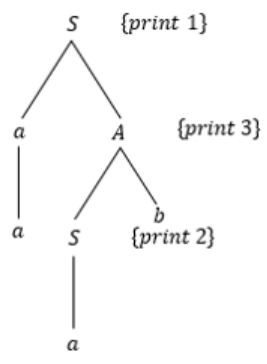Question 57

Consider a computer system with 40-bit virtual addressing and page size of sixteen kilobytes. If the computer system has a one-level page table per process and each page table entry requires 48 bits, then the size of the per-process page table is __________ megabytes.

 A 384 MB B 385 MB C 386 MB D 387 MB
Operating-Systems       Paging
Question 57 Explanation:
From the above question, we got the following information.
Size of memory = 240 and Page size = 16 KB = 214
No. of pages = size of Memory / page size = 240/ 214 = 226
Size of page table = 226 * 48 / 8 bytes = 26 * 6 MB = 384 MB
 Question 58

Consider a disk queue with requests for I/O to blocks on cylinders 47, 38, 121, 191, 87, 11, 92, 10. The C-LOOK scheduling algorithm is used. The head is initially at cylinder number 63, moving towards larger cylinder numbers on its servicing pass. The cylinders are numbered from 0 to 199. The total head movement (in number of cylinders) incurred while servicing these requests is ___________.

 A 346 B 347 C 348 D 349
Operating-Systems       Disk-Scheduling
Question 58 Explanation:
From the question, we got the following information.
I/O to blocks on cylinders 47, 38, 121, 191, 87, 11, 92, 10.
C-LOOK scheduling algorithm is used.
Head is initially at cylinder number 63.
First start from 63
63 → 87 → 92 → 121 → 191 = 24 + 5 + 29 + 70 movements = 128
191 → 10 movements = 181
10 → 11 → 38 → 47 = 1 + 27 + 9 movements = 37
Total = 128 + 181 + 37 = 346
 Question 59

Consider a computer system with ten physical page frames. The system is provided with an access sequence (a1,a2,…,a20,a1,a2,…,a20), where each ai is a distinct virtual page number. The difference in the number of page faults between the last-in-ﬁrst-out page replacement policy and the optimal page replacement policy is ___________.

 A 1 B 2 C 3 D 4
Operating-Systems       Page-Replacement-Algorithm
Question 59 Explanation:
We have to calculate the difference between the last-in-first-out page replacement policy and the optimal page replacement policy.
First we can consider LIFO (Last In First Out) →
a1 to a10 will result in page faults = 10 page faults,
Then a11 will replace a10 (last in is a10), a12 replace a11 and ...till a20 = 10 page faults and a20 will be top of stack and a9…a1 are remained as such.
Then a1 to a9 are already there.
So, 0 page faults from a1 to a9.
a10 will replace a20, a11 will replace a10 and so on = So 11 page faults.
So total faults will be 10+10+11 = 31.
Second Optimal Page Replacement Policy →
a1 to a10 = 10 page faults,
a11 will replace a10 because among a1 to a10, a10 will be used later, a12 will replace a11 and so on = 10 page faults.
a20 will be top of stack and a9…a1 are remained as such.
a1 to a9 = 0 page fault. a10 will replace a1 because it will not be used afterwards and so on, a10 to a19 will have 10 page faults.
So no page fault for a20.
Total = 10+ 10 +10 = 30.
So the difference between LIFO - Optimal = 1
 Question 60

Consider the following proposed solution for the critical section problem. There are n processes: P0...P(n-1). In the code, function pmax returns an integer not smaller than any of its arguments. For all i, t[i] is initialized to zero.

```Code for Pi:

do {

c[i]=1; t[i] = pmax(t,...,t[n-1])+1; c[i]=0;
for every j ≠ i in {0,...,n-1} {
while (c[j]);
while (t[j] != 0 && t[j]<=t[i]);
}
Critical Section;
t[i]=0;
Remainder Section;
} while (true);
```

Which one of the following is TRUE about the above solution?

 A At most one process can be in the critical section at any time B The bounded wait condition is satisﬁed C The progress condition is satisﬁed D It cannot cause a deadlock
Operating-Systems       Process-Synchronization
Question 60 Explanation:
We will check the four options one by one.
Based on the above code option B, C and D are not satisfied.
We can see that while (t[j] != 0 && t[j] <= t[i]);
because of this condition deadlock is possible when t[j] = = t[i].
Because Progress == no deadlock as no one process is able to make progress by stopping other process.
Bounded waiting is also not satisfied.
In this case both deadlock and bounded waiting to be arising from the same reason as if t[j] = = t[i] is possible then starvation is possible means infinite waiting.
Mutual exclusion is satisfied.
All other processes j started before i must have value of t[j]) < t[i] as function pMax() return a integer not smaller than any of its arguments.
So if anyone out of the processes j have positive value will be executing in its critical section as long as the condition t[j] > 0 && t[j] <= t[i] within while will persist.
And when this j process comes out of its critical section, it sets t[j] = 0; and next process will be selected in for loop.
So, when i process reaches to its critical section none of the processes j which started earlier before process i is in its critical section.
This ensure that only one process is executing its critical section at a time.
 Question 61

Consider the following two phase locking protocol. Suppose a transaction T accesses (for read or write operations), a certain set of objects{O1,...,Ok}. This is done in the following manner:

Step1. T acquires exclusive locks to O1,...,Ok in increasing order of their addresses.
Step2. The required operations are performed.
Step3. All locks are released.

This protocol will

 A guarantee serializability and deadlock-freedom B guarantee neither serializability nor deadlock-freedom C guarantee serializability but not deadlock-freedom D guarantee deadlock-freedom but not serializability
Database-Management-System       Two Phase Locking protocol
Question 61 Explanation:
Conservative 2PLP:
In conservative 2PL protocol, a transaction has to lock all the items before the transaction begins execution.
• Ensure serializability.
The given scenario (Step1, Step2 and Step3) is conservative 2PLP.
 Question 62

Consider that B wants to send a message m that is digitally signed to A. Let the pair of private and public keys for A and B be denoted by Kx- and Kx+ for x = A,B, respectively. Let Kx(m)  represent the operation of encrypting m with a key Kx and H(m) represent the message digest. Which one of the following indicates the CORRECT way of sending the message m along with the digital signature to A?

 AB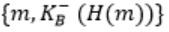C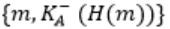DComputer-Networks       Security
Question 62 Explanation:
Digital signatures are electronic signatures which ensure the integrity, non-repudiation and authenticity of message.
Message digest is a hash value generated by applying a function on it.
Message digest is encrypted using private key of sender, so it can only be decrypted by public key of sender.
This ensures that the message was sent by the known sender.
Message digest is sent with the original message to the receiving end, where hash function is used on the original message and the value generated by that is matched with the message digest.
This ensures the integrity and thus, that the message was not altered.
Digital signature uses private key of the sender to sign message digest.Question 63

An IP datagram of size 1000 bytes arrives at a router. The router has to forward this packet on a link whose MTU (maximum transmission unit) is 100 bytes. Assume that the size of the IP header is 20 bytes.

The number of fragments that the IP datagram will be divided into for transmission is _________.

 A 13 B 14 C 15 D 16
Computer-Networks       IPv4-and-Fragmentation
Question 63 Explanation:Size of Datagram (L) = 1000 bytes
MTU = 100 bytes
Size of IP header = 20 bytes
Size of Data that can be transmitted in one fragment (payload) = 100 – 20 = 80 bytes
Size of Data to be transmitted = Size of Datagram – size of header = 1000 – 20 = 980 bytes
No. of fragments required = ⌈980/80⌉ = 13
 Question 64

For a host machine that uses the token bucket algorithm for congestion control, the token bucket has a capacity of 1 megabyte and the maximum output rate is 20 megabytes per second. Tokens arrive at a rate to sustain output at a rate of 10 megabytes per second. The token bucket is currently full and the machine needs to send 12 megabytes of data. The minimum time required to transmit the data is seconds _________.

 A 1.1 sec B 1.2 sec C 1.3 sec D 1.4 sec
Computer-Networks       Token-Bucket
Question 64 Explanation:
According to the token bucket algorithm, the minimum time required sending 1 MB of data or the maximum rate of data transmission is given by:
S = C / (M - P)
Where,
M = Maximum output rate,
C = capacity of the bucket,
P = Rate of arrival of a token,
Given, M=20 Mb, C=1Mbps, P=10 Mbps
Therefore, S= 1 Mb / (20-10) Mbps = 1/10 = 0.1 sec
Since, the bucket is initially full, it already has 1 Mb to transmit so it will be transmitted instantly.
So, we are left with only (12 – 1) Mb, i.e. 11 Mb of data to be transmitted.
Therefore, time required to send the 11 MB will be 11 * 0.1 = 1.1 sec
 Question 65

A sender uses the Stop-and-Wait ARQ protocol for reliable transmission of frames. Frames are of size 1000 bytes and the transmission rate at the sender is 80 Kbps (1Kbps = 1000 bits/second). Size of an acknowledgement is 100 bytes and the transmission rate at the receiver is 8 Kbps. The one-way propagation delay is 100 milliseconds.

Assuming no frame is lost, the sender throughput is _________ bytes/second.

 A 2500 B 2501 C 2502 D 2503
Computer-Networks       Stop-and-Wait-ARQ
Question 65 Explanation:
Given,
Frame size (L) =1000 bytes
Sender side bandwidth (BS) = 80 kbps = 10 * 103 bytes/sec
Acknowledgement size (LA) =100 bytes
Receiver side bandwidth (BR) = 8 kbps = 1 * 103 bytes/sec
Propagation delay (Tp) =100 ms
By formula:
Transmission delay (Tt ) = L/BS = 1000 bytes / 10 * 103 bytes/sec = 100 ms
Acknowledge delay (Tack ) = LA / BR = 100 bytes / 1 * 103 bytes/sec = 100 ms
Total cycle time = Tt + 2 * Tp + Tack = 100 ms + 2 * 100 ms + 100 ms = 400 ms
Efficiency (η) = Tt / Total cycle time = 100 ms / 400 ms = 1 / 4 = 0.25
Throughput = Efficiency (η) * Bandwidth (BS) = 0.25 * 10 *103 bytes/s = 2500 bytes/second
There are 65 questions to complete.